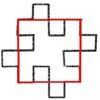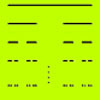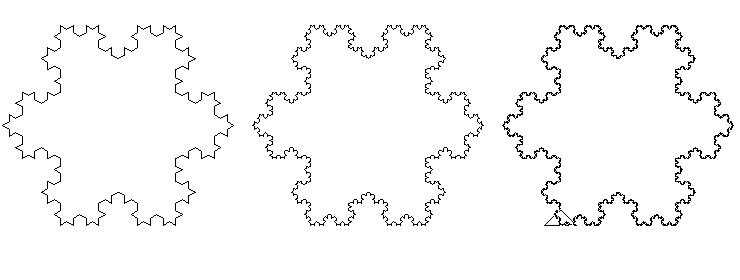#### You may also like### Sierpinski Triangle

What is the total area of the triangles remaining in the nth stage of constructing a Sierpinski Triangle? Work out the dimension of this fractal.### Squareflake

A finite area inside and infinite skin! You can paint the interior of this fractal with a small tin of paint but you could never get enough paint to paint the edge.### How Many Elements Are There in the Cantor Set?

This article gives a proof of the uncountability of the Cantor set.

# Von Koch Curve

##### Age 16 to 18 Challenge Level:

The Von Koch curve is a fractal. The rule for generating this curve is to start with an equilateral triangle and to replace each line segment by a zig-zag curve (a generator) made up of $4$ copies of the line segment it replaces, each reduced to one third of the original length.

Click on the red button below to see the first six stages of the infinite process for generating the Von Koch curve.

Full Screen Version

The original equilateral triangle has side $1$ unit. Work out the length of this curve in the first few stages and the length of the fractal curve formed when the process goes on indefinitely.

Now suppose you made a poster for your classroom with coloured paper by drawing an equilateral triangle for Stage 0 and then cutting smaller equilateral triangles and sticking them on the edge. What is the total area of all the triangles you would stick on one edge if you could continue the process indefinitely to make the Von Koch curve? So what is the area inside the Von Koch curve?

Find the dimension of the Von Koch curve using the formula $n=m^d$, where where $n$ is the number of self similar pieces in the generator and $m$ is the magnification factor.

The diagrams below show Stages 0 to 5 in the evolution of the Von Koch curve. The Logo program for drawing this fractal is given in the Notes.

See First Forward for a ten part series giving an introduction to Logo programming for beginners.